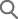• 회원님의 아이디와 패스워드를 입력해 주세요.
• 회원이 아니시면 아래 [회원가입]을 눌러 회원가입을 해주시기 바랍니다.

아이디 저장

HONGGIDONG
사용 가능한 회원 아이디 입니다.

honggildong@naver.com
사용 가능한 E-mail 주소 입니다.

• * 도로명, 건물명, 지번에 대해 통합검색이 가능합니다.
(예: 반포대로 58, 국립중앙박물관, 삼성동 25)
• * 빠른 검색을 위해 주소, 도로명, 건물명, 기타시설물명(지하철역사명/기타시설물 등)을 정확하게 입력하
여 주세요.
• * 약식으로 검색하시는 경우 검색이 제대로 되지 않을 수 있습니다.
(예: 반포, 국중, 삼성)• 아이디/비밀번호를 잊으셨나요? 아래 항목을 정확히 입력해 주시기 바랍니다.

비밀번호 찾기

아이디

성명

E-mail

학술자료 검색

### Study on Out-of-Sample Predictability of the Monetary Model for the Exchange Rate Using Long Span Data

To examine the common assumption that the monetary fundamentals are stationary, we test the monetary model of exchange rate determination. In specific, we test whether nominal exchange rates are cointegrated with the monetary fundamentals, using data spanning from January 1971 to March 2011. The results show that the Phillips and Ouliaris test fails to reject the null of no cointegration while we reject the null hypothesis of no cointegration with Johansen’s trace test. We also explore the out-of-sample forecasting performance of the long-horizon regression of exchange rate returns on the deviation of the log exchange rate from the monetary fundamentals. For the sample of data, the DOLS estimation does not yield cointegrating coefficient estimates that accord with the theoretical values implied by the monetary model of exchange rate determination. This may bring about critical results asserting that there is no evidence of exchange rate predictability based on the monetary fundamentals in the sample. Despite the use of long span data, no evidence is found in favor of the monetary exchange rate model using the Johansen procedures. We compare the out-of-sample forecasting performance of the monetary model and confirm that forecasting power of the monetary model is insignificant.
• Ho-Jin Lee
To examine the common assumption that the monetary fundamentals are stationary, we test the monetary model of exchange rate determination. In specific, we test whether nominal exchange rates are cointegrated with the monetary fundamentals, using data spanning from January 1971 to March 2011. The results show that the Phillips and Ouliaris test fails to reject the null of no cointegration while we reject the null hypothesis of no cointegration with Johansen’s trace test. We also explore the out-of-sample forecasting performance of the long-horizon regression of exchange rate returns on the deviation of the log exchange rate from the monetary fundamentals. For the sample of data, the DOLS estimation does not yield cointegrating coefficient estimates that accord with the theoretical values implied by the monetary model of exchange rate determination. This may bring about critical results asserting that there is no evidence of exchange rate predictability based on the monetary fundamentals in the sample. Despite the use of long span data, no evidence is found in favor of the monetary exchange rate model using the Johansen procedures. We compare the out-of-sample forecasting performance of the monetary model and confirm that forecasting power of the monetary model is insignificant.
Exchange Rate; Monetary Model; Cointegration; Unit Root; Out-of-Sample Forecast